# Review: MemNet — A Persistent Memory Network for Image Restoration (Denoising & Super Resolution & JPEG Deblocking)

## DenseNet-like Network, Outperforms SRCNN, ARCNN, RED-Net, VDSR, DRCN, DnCNN, LapSRN, DRRN

In this story, a very deep persistent memory network (MemNet), by Nanjing University of Science and Technology, and Michigan State University, is reviewed. In MemNet:

• A memory block is introduced, consisting of a recursive unit and a gate unit, to explicitly mine persistent memory through an adaptive learning process.
• The recursive unit learns multi-level representations of the current state under different receptive fields. The representations and the outputs from the previous memory blocks are concatenated and sent to the gate unit.
• The gate unit adaptively controls how much of the previous states should be reserved, and decides how much of the current state should be stored.

This is a paper in 2017 ICCV with over 300 citations for image denoising, super resolution & JPEG deblocking. (Sik-Ho Tsang @ Medium)

# Outline

1. Network Architecture
2. Memory Block
3. Multi-Supervised MemNet
4. Experimental Results

# 1. Network Architecture

• MemNet consists of three parts: a feature extraction net FENet, multiple stacked memory blocks and finally a reconstruction net ReconNet.
• FENet: Specifically, a convolutional layer is used in FENet to extract the features from the noisy or blurry input image:
• where fext denotes the feature extraction function and B0 is the extracted feature to be sent to the first memory block.
• Stacked Memory Blocks: Supposing M memory blocks are stacked to act as the feature mapping:
• where Mm denotes the m-th memory block function, Bm-1 and Bm are the input and output of the m-th memory block respectively.
• ReconNet: A convolutional layer is used to reconstruct the residual image.
• where frec denotes the reconstruction function and D denotes the function of our basic MemNet.
• MSE is used as loss function:
• (The network somehow is quite similar to DenseNet.)

# 2. Memory Block

• The memory block contains a recursive unit and a gate unit.

## 2.1. Recursive Unit

• Recursive Unit is used to model a non-linear function that acts like a recursive synapse in the brain.
• A residual building block is used, which is introduced in ResNet. A residual building block in the m-th memory block is formulated as:
• where Hr-1m, Hrm are the input and output of the r-th residual building block respectively. Wm is the weight set to be learned and R denotes the function of residual building block.
• Each residual function contains two convolutional layers with the pre-activation structure, originated in Pre-Activation ResNet:
• where τ denotes the activation function, including batch normalization followed by ReLU.
• Then, several recursions are recursively learned to generate multi-level representations under different receptive fields.
• Supposing there are R recursions in the recursive unit, the r-th recursion in recursive unit can be formulated:
• These representations within the memory blocks are concatenated as the short-term memory, similar to DenseNet:
• In addition, the long-term memory coming from the previous memory blocks can be constructed as:
• Finally, both short-term memory and long-term memory are concatenated and input to the gate unit:

## 2.2. Gate Unit

• Gate Unit is used to achieve persistent memory through an adaptive learning process. A 1×1 convolutional layer is used to accomplish the gating mechanism that can learn adaptive weights for different memories:
• where fgatem and Bm denote the function of the 1×1 convolutional layer (parameterized by Wgatem) and the output of the m-th memory block, respectively.
• As a result, the weights for the long-term memory controls how much of the previous states should be reserved, and the weights for the short-term memory decides how much of the current state should be stored.
• Therefore, the formulation of the m-th memory block can be written as:

# 3. Multi-Supervised MemNet

• To further explore the features at different states, the output of each memory block is sent to the same reconstruction net ^ frec to generate.
• All ym, where m from 1 to M, are all intermediate predictions.
• The final prediction output is the weighted average of ym where the weight is also learnt.
• Thus, the loss function of the multi-supervised MemNet become:

# 4. Experimental Results

## 4.1. Dataset

• For image denoising, 300 images from the Berkeley Segmentation Dataset (BSD) is used, known as the train and val sets, to generate image patches as the training set.
• Two popular benchmarks, a dataset with 14 common images and the BSD test set with 200 images, are used for evaluation.
• Gaussian noise with one of the three noise levels (σ = 30, 50 and 70) is added to the clean patch.
• For Single Image Super Resolution (SISR), a training set of 291 images is used where 91 images are from Yang et al.  and other 200 are from BSD train set.
• For testing, four benchmark datasets, Set5, Set14, BSD100 and Urban100 are used. Three scale factors are evaluated, including ×2, ×3 and ×4.
• The input LR image is generated by first bicubic downsampling and then bicubic upsampling the HR image with a certain scale.
• For JPEG deblocking, the same training set for image denoising is used.
• Classic5 and LIVE1 are adopted as the test datasets.
• Two JPEG quality factors are used, i.e., 10 and 20, and the JPEG deblocking input is generated by compressing the image with a certain quality factor using the MATLAB JPEG encoder.

## 4.2. Network

• Two 80-layer MemNet networks, the basic and the multi-supervised versions.
• In both architectures, 6 memory blocks, each contains 6 recursions, are constructed (i.e., M6R6).
• Specifically, in multi-supervised MemNet, 6 predictions are generated and used to compute the final output.
• All convolutional layer has 64 filters. Except the 1×1 convolutional layers in the gate units, the kernel size of other convolutional layers is 3×3.

## 4.3. Ablation Study

4.3.1. Long Term & Short Term Connections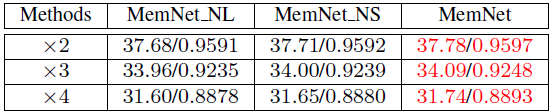Ablation study on effects of long-term and short-term connections
• MemNet_NL: removes the long-term connections.
• MemNet_NS: removes the short-term connections.
• Long-term dense connections are very important since MemNet significantly outperforms MemNet NL.
• Further, MemNet achieves better performance than MemNet NS, which reveals the short-term connections are also useful for image restoration but less powerful than the long-term connections.

4.3.2. Gate Unit Analysis

• A weight norm is adopted as an approximate for the dependency of the current layer on its preceding layers, which is calculated by the corresponding weights from all filters w.r.t. each feature map:
• Basically, the larger the norm is, the stronger dependency it has on this particular feature map.
• Three observations are found by authors:
• (1) Different tasks have different norm distributions.
• (2) The average and variance of the weight norms become smaller as the memory block number increases.
• (3) In general, the short-term memories from the last recursion in recursive unit (the last 64 elements in each curve) contribute most than the other two memories, and the long-term memories seem to play a more important role in late memory blocks to recover useful signals than the short-term memories from the first R-1 recursions.
• Four network structures are tested: M4R6, M6R6, M6R8 and M10R10, which have the depth 54, 80, 104 and 212, respectively.
• The above table shows the SISR performance of these networks on Set5 with scale factor ×3. It verifies deeper is still better and the proposed deepest network M10R10 achieves 34.23 dB, with the improvement of 0.14 dB compared to M6R6.
• But M6R6 is still used for SOTA comparison as below.

## 4.4. SOTA Comparison

4.4.1. Parameters and Complexity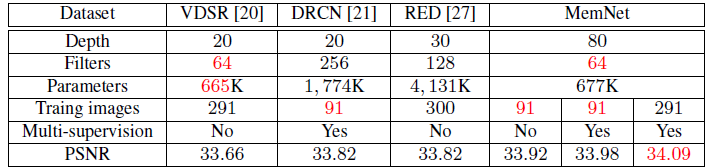SISR comparisons with start-of-the-art networks for scale factor ×3 on Set5.
• Using the fewest training images (91), filter number (64) and relatively few model parameters (667K), the basic MemNet already achieves higher PSNR than the prior networks.
• Keeping the setting unchanged, the multi-supervised MemNet further improves the performance.
• With more training images (291), the MemNet significantly outperforms the state of the arts.
• The above figuure shows the PSNR of different intermediate predictions in MemNet (e.g., MemNet_M3 denotes the prediction of the 3rd memory block) for scale ×3 on Set5.
• MemNet already achieve comparable result as DRCN at the 3rd prediction using much fewer parameters, and significantly outperforms the state of the arts by slightly increasing model complexity.

## 4.4.2. Image Denoising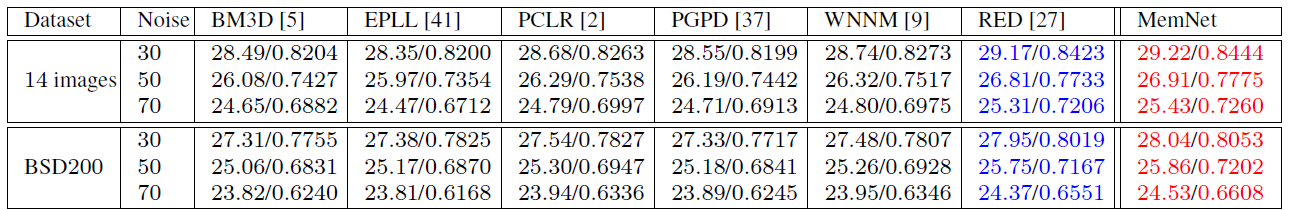Average PSNR/SSIMs for noise level 30, 50 and 70 on 14 images and BSD200
• The MemNet achieves the best performance on all cases, outperforms RED-Net.

## 4.4.3. Super Resolution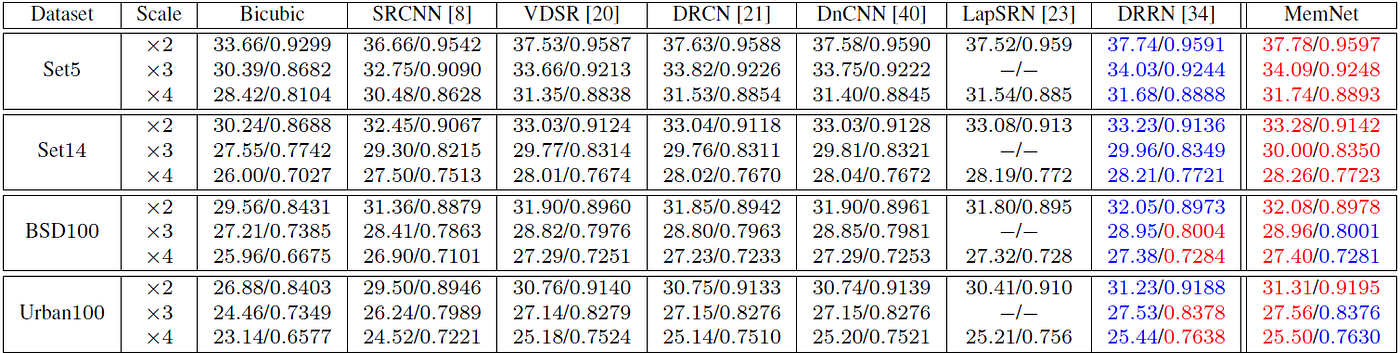Average PSNR/SSIMs for scale factor ×2, ×3 and ×4 on datasets Set5, Set14, BSD100 and Urban100

## 4.4.4. JPEG Deblocking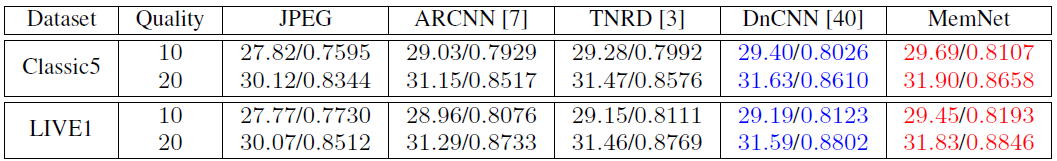Average PSNR/SSIMs for quality factor 10 and 20 on datasets Classic5 and LIVE1
• The network significantly outperforms the other methods, such as ARCNN and DnCNN.

During the days of coronavirus, I hope to write 30 stories in this month to give myself a small challenge. This is the 28th story in this month. Thanks for visiting my story…

## Reference

[2017 ICCV] [MemNet]
MemNet: A Persistent Memory Network for Image Restoration

## Super Resolution

[SRCNN] [FSRCNN] [VDSR] [ESPCN] [RED-Net] [DnCNN] [DRCN] [DRRN] [LapSRN & MS-LapSRN] [MemNet] [SRDenseNet] [SRGAN & SRResNet] [EDSR & MDSR][SR+STN]

## Codec Filtering

JPEG: [ARCNN] [RED-Net] [DnCNN] [Li ICME’17] [MemNet]
HEVC:[Lin DCC’16] [IFCNN] [VRCNN] [DCAD] [MMS-net] [DRN] [Lee ICCE’18] [DS-CNN] [RHCNN] [VRCNN-ext] [S-CNN & C-CNN]
VVC: [Lu CVPRW’19] [Wang APSIPA ASC’19]

--

--